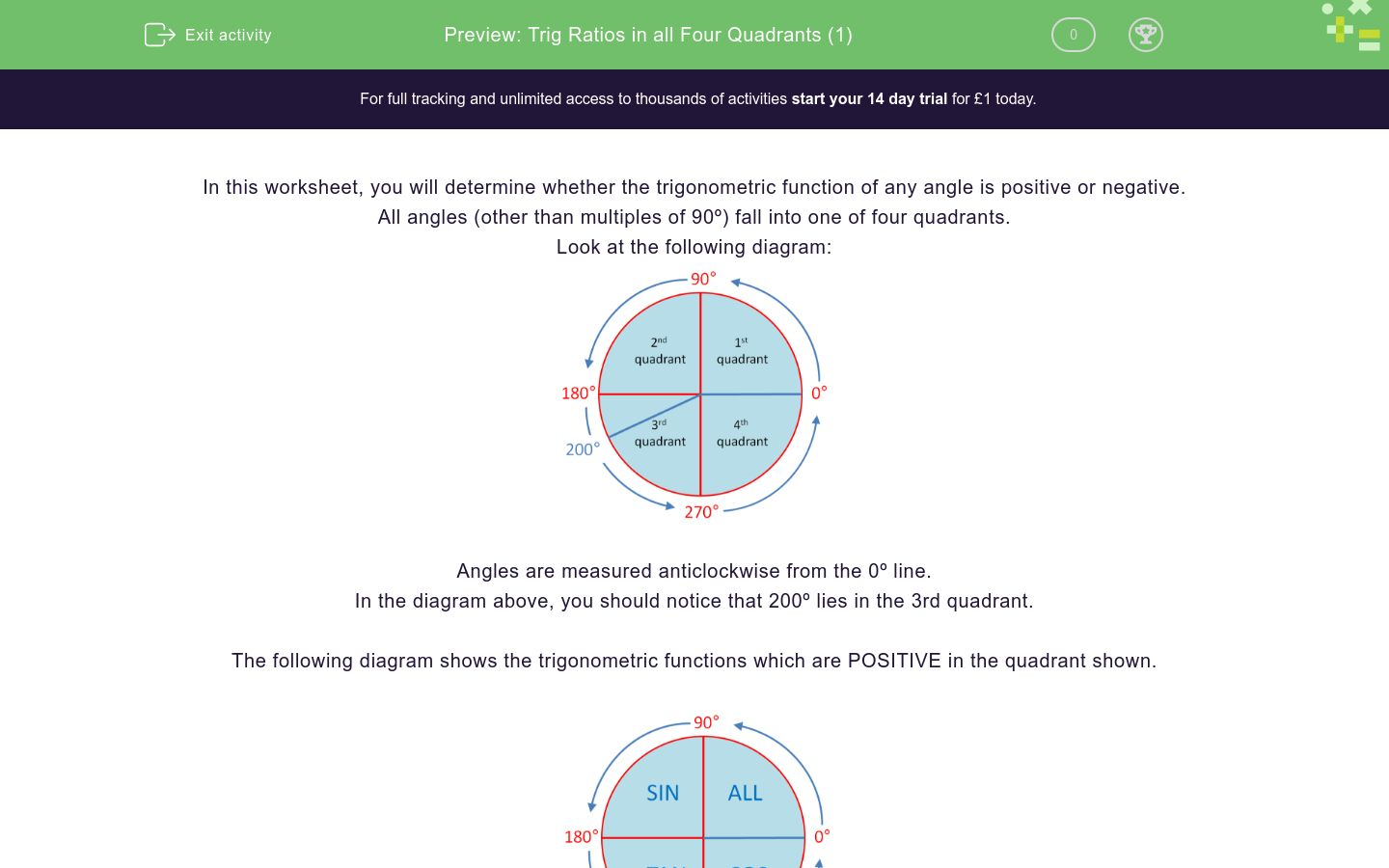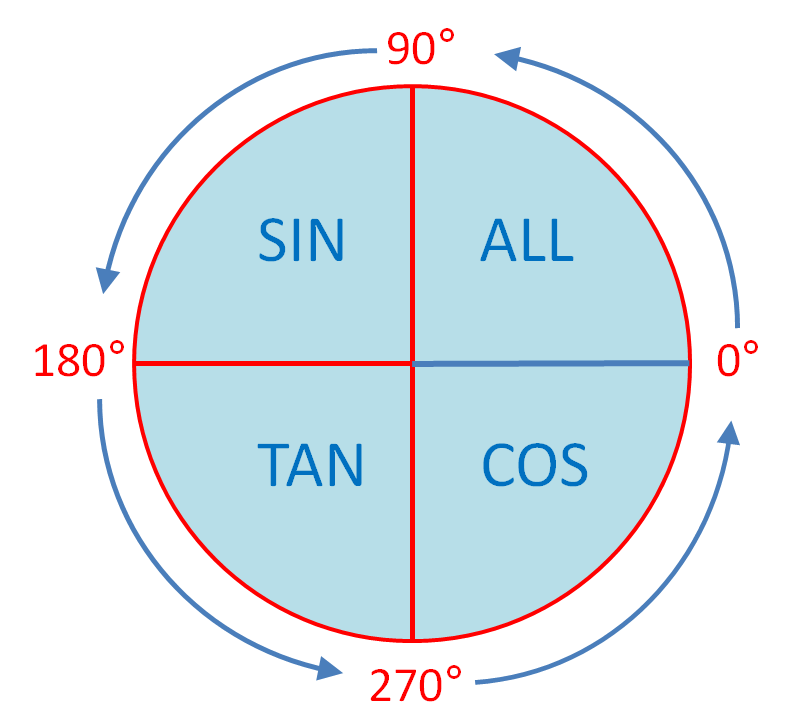# Trig Ratios in all Four Quadrants (1)

In this worksheet, students state whether a trigonometric function is positive or negative by looking at the quadrant in which the angle lies.Key stage:  KS 4

Curriculum topic:  Ratio, Proportion and Rates of Change

Curriculum subtopic:  Compare Lengths, Areas and Volumes Using Ratio Notation/Scale Factors

Difficulty level:### QUESTION 1 of 10

In this worksheet, you will determine whether the trigonometric function of any angle is positive or negative.

All angles (other than multiples of 90º) fall into one of four quadrants.

Look at the following diagram:Angles are measured anticlockwise from the 0º line.

In the diagram above, you should notice that 200º lies in the 3rd quadrant.

The following diagram shows the trigonometric functions which are POSITIVE in the quadrant shown.Remember ASTC (ALL SIN TAN COS or "All Silly Tom Cats")

Example 1

State which of sin 200º, cos 200º or tan 200º is positive.

Draw 200º on the ASTC circle.It shows that the only trig ratio that is positive is tan 200º.

Example 2

State which of sin 300º, cos 300º or tan 300º is positive.

Draw 300º on the ASTC circle.It shows that the only trig ratio that is positive is cos 300º.

State which of sin 220º, cos 220º or tan 220º is positive.

sin 220°

cos 220°

tan 220°

State which of sin 170º, cos 170º or tan 170º is positive.

sin 170°

cos 170°

tan 170°

State which of sin 70º, cos 70º or tan 70º is positive.

sin 70°

cos 70°

tan 70°

State which of sin 340º, cos 340º or tan 340º is positive.

sin 340°

cos 340°

tan 340°

State which of sin 100º, cos 100º or tan 100º is positive.

sin 100°

cos 100°

tan 100°

State which of sin 153º, cos 153º or tan 153º is positive.

sin 153°

cos 153°

tan 153°

State which of sin 245º, cos 245º or tan 245º is positive.

sin 245°

cos 245°

tan 245°

State which of sin 5º, cos 5º or tan 5º is positive.

sin 5°

cos 5°

tan 5°

State which of sin 365º, cos 365º or tan 365º is positive.

sin 365°

cos 365°

tan 365°

State which of sin 690º, cos 690º or tan 690º is positive.

sin 690°

cos 690°

tan 690°

• Question 1

State which of sin 220º, cos 220º or tan 220º is positive.

tan 220°
EDDIE SAYS
220° is in the 3rd quadrant where tan is positive.
• Question 2

State which of sin 170º, cos 170º or tan 170º is positive.

sin 170°
EDDIE SAYS
170° is in the 2nd quadrant where sin is positive.
• Question 3

State which of sin 70º, cos 70º or tan 70º is positive.

sin 70°
cos 70°
tan 70°
EDDIE SAYS
70° is in the 1st quadrant where all trig ratios are positive.
• Question 4

State which of sin 340º, cos 340º or tan 340º is positive.

cos 340°
EDDIE SAYS
340° is in the 4th quadrant where cos is positive.
• Question 5

State which of sin 100º, cos 100º or tan 100º is positive.

sin 100°
EDDIE SAYS
100° is in the 2nd quadrant where sin is positive.
• Question 6

State which of sin 153º, cos 153º or tan 153º is positive.

sin 153°
EDDIE SAYS
153° is in the 2nd quadrant where sin is positive.
• Question 7

State which of sin 245º, cos 245º or tan 245º is positive.

tan 245°
EDDIE SAYS
245° is in the 3rd quadrant where tan is positive.
• Question 8

State which of sin 5º, cos 5º or tan 5º is positive.

sin 5°
cos 5°
tan 5°
EDDIE SAYS
5° is in the 1st quadrant where all trig ratios are positive.
• Question 9

State which of sin 365º, cos 365º or tan 365º is positive.

sin 365°
cos 365°
tan 365°
EDDIE SAYS
365° is in the 1st quadrant where all trig ratios are positive.
• Question 10

State which of sin 690º, cos 690º or tan 690º is positive.

cos 690°
EDDIE SAYS
690° is in the 4th quadrant where cos is positive.
---- OR ----

Sign up for a £1 trial so you can track and measure your child's progress on this activity.

### What is EdPlace?

We're your National Curriculum aligned online education content provider helping each child succeed in English, maths and science from year 1 to GCSE. With an EdPlace account you’ll be able to track and measure progress, helping each child achieve their best. We build confidence and attainment by personalising each child’s learning at a level that suits them.

Start your £1 trial